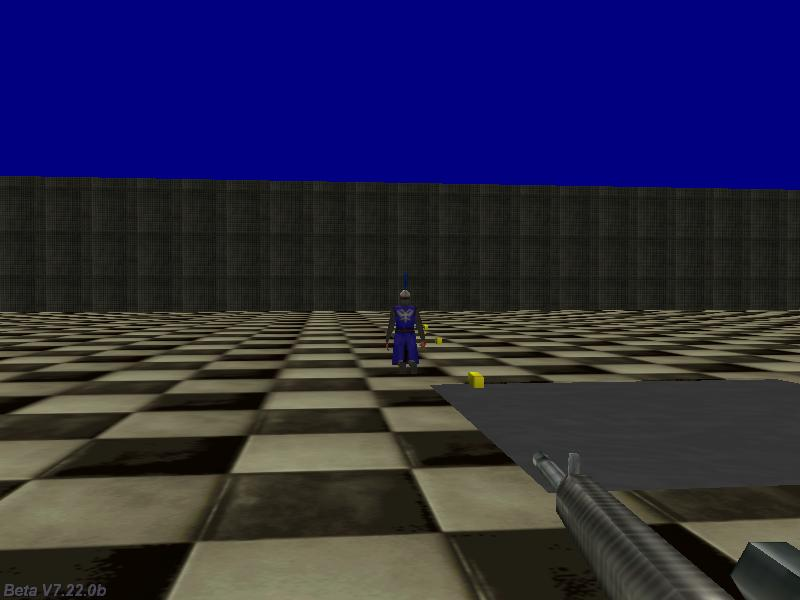Questions from the forum
 Q: I want to display a string just like a typewriting machine does, so that each letter or character is displayed after a little break. A: Here's an example.   STRING* temp_str = "#100"; STRING* backup_str = "#100"; STRING* typewriter_str = "Hello! This is a typewriter test!";   SOUND* typewriter_wav = "typewriter.wav";   TEXT* typewriter_txt = {                pos_x = 20;                pos_y = 20;                string(temp_str);        flags = VISIBLE; }   function init_startup() // call this function whenever you need to {          wait (-1); // wait until the level is loaded        var i = str_len(typewriter_str);          while(i > 0)          {                        i -= 1;                        str_cpy(backup_str, typewriter_str);                str_trunc(backup_str, i);                str_cpy(temp_str, backup_str);                snd_play(typewriter_wav, 60, 0);                wait (-0.3);        } }     Q: I would need a lite-C camera that always shows the player from its side. A: There you go.   action player_and_cam() // simple player and camera code {        var walk_percentage;        var stand_percentage;        player = my; // I'm the player        while (1)        {                // move the player using the "W", "S", "A" and "D" keys; "10" = movement speed,                c_move (my, vector(10 * (key_w - key_s) * time_step, 0, 0), nullvector, GLIDE);                my.pan += 6 * (key_a - key_d) * time_step; // "6" = rotating speed                // place the camera 0 quants in front of the player, 200 quants sideways and 25 quants above player's origin                vec_set (camera.x, vector(0, 200, 25));                vec_rotate (camera.x, player.pan);                vec_add (camera.x, player.x);                camera.pan = player.pan - 90; // make the camera look at the player from a sideview perspective                if (key_w + key_s) // the player is moving?                {                        walk_percentage += 5 * time_step;                        ent_animate(my, "walk", walk_percentage, ANM_CYCLE);                        stand_percentage = 0;                }                else // the player is standing still (or rotating)                {                        stand_percentage += 1.5 * time_step;                        ent_animate(my, "stand", stand_percentage, ANM_CYCLE);                        walk_percentage = 0;                }                wait (1);        } }     Q: When a player gets a high score, I'd like to be able to prompt him to type in a name and then write it to a file. So far, I haven't discovered any Lite-C mechanism for doing this. A: Here's an example that generates a random number and if it is bigger than the "high score" it writes its value, as well as player's name, to a file.   var high_score = 0; var current_score = 0;   STRING* name_str = "#50"; // allow the name to have up to 50 characters STRING* input_str = "#50";   TEXT* name_txt = {        pos_x = 10;        pos_y = 10;        string(name_str);        flags = VISIBLE; }   TEXT* input_txt = {        pos_x = 10;        pos_y = 550;        string(input_str);        flags = VISIBLE; }   PANEL* score_txt = {        pos_x = 10;        pos_y = 10;        digits(300, 10, 4, *, 1, high_score);        digits(300, 300, 4, *, 1, current_score);        flags = VISIBLE; }   function random_number() {        var filehandle;        current_score = integer(random(100));        if (current_score > high_score) // a new high score was achieved?        {                high_score = current_score;                str_cpy(input_str, "New high score! Please type a name!");                wait (-3); // display the message for 3 seconds                str_cpy(input_str, "#50"); // reset the string                inkey(input_str); // now let's input player's name                str_cpy(name_str, input_str); // let's update the high score name on the screen as well                filehandle = file_open_write("highscore.txt"); // overwrite the existing highscore.txt file (if any)                file_str_write(filehandle, input_str);                file_asc_write (filehandle, 13); // write the following value on a separate line                file_asc_write (filehandle, 10); // using asc(13) = carriage return + asc(10) = line feed                file_var_write(filehandle, high_score);                file_close(filehandle);        } }   function score_startup() {        var filehandle;        filehandle = file_open_read("highscore.txt");        if (filehandle) // the file exists?        {                file_str_read(filehandle, name_str);                high_score = file_var_read(filehandle);        }        else // the highscore.txt file doesn't exist        {                str_cpy(name_str, "None");                high_score = 0;        }        on_r = random_number; // generates a random number every time when the player presses the "R" key }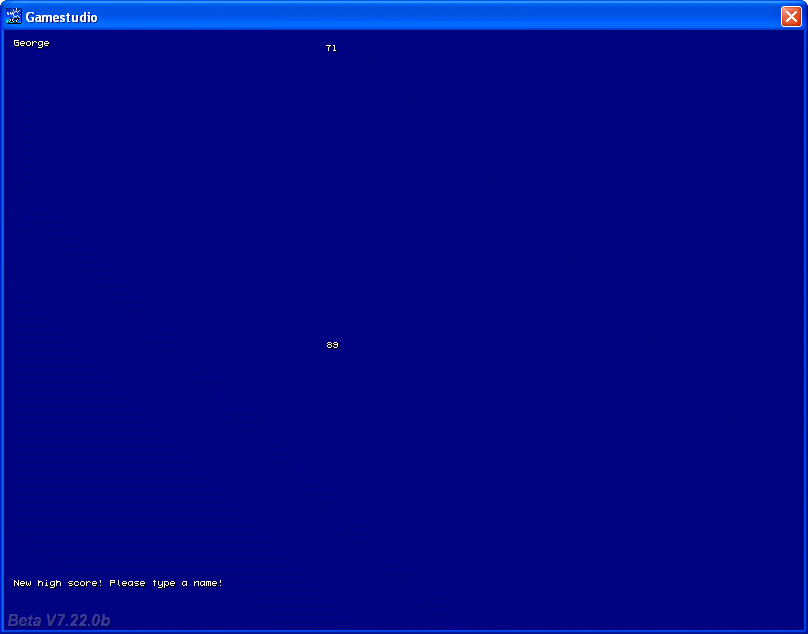Q: I know that the goto instruction doesn't work in lite-C. Can you show me a good example on how to replace goto in lite-C? A: Here's a goto lite-C example that works fine. Don't forget that the goto label must be placed in the same function, between two instructions. Please remember that using goto instructions will complicate the things for you, as well as for the other potential programmers from your team.   function goto_startup() // simple goto example {        randomize();        var i = random(1);        if (i < 0.5)                goto beep_once;        else                goto beep_twice;        return; beep_once:        beep();        return; beep_twice:        beep(); beep(); }     Q: I would like to have a looping sound fade in / out as a player gets closer / farther to it. A: You can get what you want by simply adding a sound to the level in Wed; however, if you need more control over it, you can use something like this.   action looping_sound() // place a small entity in Wed and attach it this action {        var sound_handle;        var sound_volume;        set (my, PASSABLE | INVISIBLE);        // make sure that the player action includes a line of code that looks like this:        // "player = my;" (without the quotes)        while (!player) {wait (1);}        var dist_to_player;        sound_handle = media_loop("mysound.wav", NULL, 1); // start with a small volume        while (1)        {                dist_to_player = vec_dist(player.x, my.x);                sound_volume = maxv(1, 5000 / (dist_to_player + 1)); // feel free to use your own formula here                media_tune(sound_handle, sound_volume, 0, 0); // tune the sound volume each and every frame                wait (1);        } }     Q: I'm making a subway and I want the doors to move along with the subway. How can I do this? A: Use the following example.   // create the subway doors as mdl entities and name them subdoor1.mdl and subdoor2.mdl function subway_door1() {        while (1)        {                vec_set(my.x, vector(-100, -40, 10));                vec_rotate(my.x, you.pan);                vec_add(my.x, you.x);                wait (1);        } }   function subway_door2() {        while (1)        {                vec_set(my.x, vector(100, -40, 10));                vec_rotate(my.x, you.pan);                vec_add(my.x, you.x);                wait (1);        } }   action my_subway() // attach this action to the subway {        ent_create("subdoor1.mdl", nullvector, subway_door1);        ent_create("subdoor2.mdl", nullvector, subway_door2);        while (1)        {                // primitive movement code; move the "real" subway using c_move, etc                // the following loops simply move the subway back and forth along the x axis (0... 1000 quants)                while (my.x < 1000)                {                        my.x += 20 * time_step;                        wait (1);                }                wait (-2);                while (my.x > 0)                {                        my.x -= 20 * time_step;                        wait (1);                }                wait (-2);        } }     Q: How do you create bullets for a gun? I know that you can ent_create them, but how do you make it so that they will always end up coming out of the gun? A: You can ent_create the bullets using an offset from player's origin or player's gun, just like I did it in this month's multiplayer workshop, or you can generate them from the desired vertex of the gun like this:   ENTITY* weapon1;   function attach_weapon1() {        weapon1 = my; // I'm the gun        set(my, PASSABLE);        while (1)        {                vec_set (my.x, vector (20, -10, 35)); // set the proper gun offset in relation to the player                vec_rotate (my.x, you.pan);                vec_add (my.x, you.x);                my.pan = you.pan;                my.tilt = camera.tilt;                wait (1);        } }   action players_code() // simple player and 1st person camera code {        player = my; // I'm the player        ent_create ("weapon1.mdl", nullvector, attach_weapon1);        while (1)        {                // move the player using the "W", "S", "A" and "D" keys; "10" = movement speed, "6" = strafing speed                c_move (my, vector(10 * (key_w - key_s) * time_step, 6 * (key_a - key_d) * time_step, 0), nullvector, GLIDE);                vec_set (camera.x, player.x); // use player's x and y for the camera as well                camera.z += 30; // place the camera 30 quants above the player on the z axis (approximate eye level)                camera.pan -= 5 * mouse_force.x * time_step; // rotate the camera around by moving the mouse                camera.tilt += 3 * mouse_force.y * time_step; // on its x and y axis                player.pan = camera.pan; // the camera and the player have the same pan angle                  wait (1);        } }     function remove_bullets() // this function runs when the bullet collides with something {        wait (1); // wait a frame to be sure (don't trigger engine warnings)        ent_remove (my); // and then remove the bullet }   function move_bullets() {        VECTOR bullet_speed; // stores the speed of the bullet        // the bullet is sensitive to impacts with entities and level blocks        my.emask = ENABLE_IMPACT | ENABLE_ENTITY | ENABLE_BLOCK;        my.event = remove_bullets; // when it collides with something, its event function (remove_bullets) will run        my.pan = weapon1.pan;        my.tilt = weapon1.tilt;        bullet_speed.x = 35 * time_step; // adjust the speed of the bullet here        bullet_speed.y = 0; // the bullet doesn't move sideways        bullet_speed.z = 0; // or up / down on the z axis        while (my) // this loop will run for as long as the bullet exists (it isn't "NULL")        {                // move the bullet ignoring its creator (weapon1)                c_move (my, bullet_speed, nullvector, IGNORE_PASSABLE | IGNORE_YOU);                wait (1);        } }   function fire_bullets() {        VECTOR bullet_pos;        // generate bullets from the 6th vertex of the weapon (get the vertex number from Med)        vec_for_vertex(bullet_pos, weapon1, 6);        ent_create("bullet.mdl", bullet_pos, move_bullets); }   function bullets_startup() {        on_mouse_left = fire_bullets; }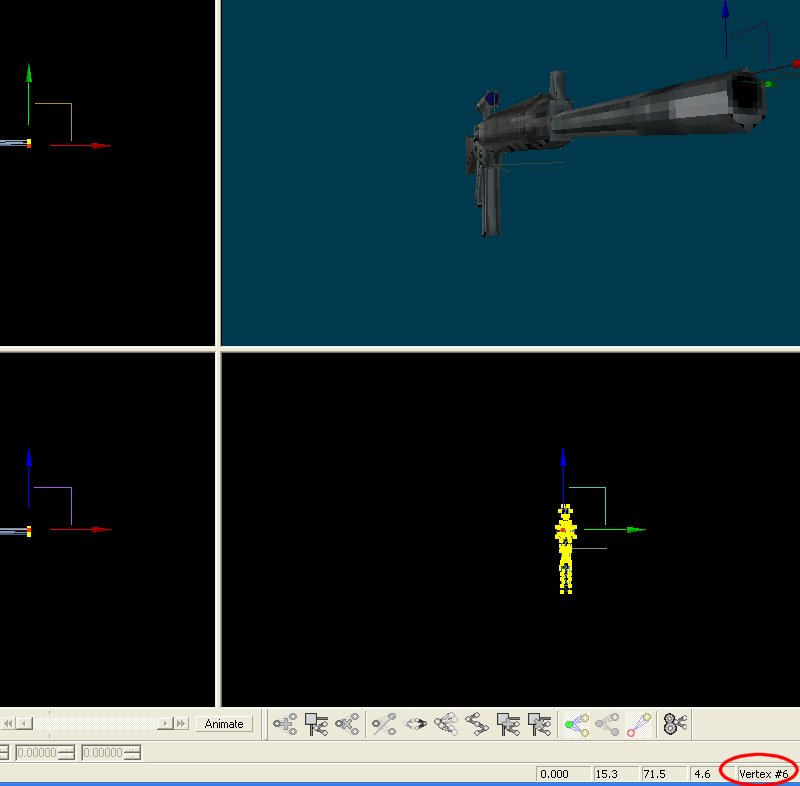Q: How do I make it so that when the bullet hits the target, the target dies or an effect triggers? A: Here's an example that does both things.   BMAP* sparks_tga = "sparks.tga";   function fade_sparks(PARTICLE *p) {        p.alpha -= 2 * time_step; // fade out the sparks        if (p.alpha < 0)                p.lifespan = 0; }   function sparks_effect(PARTICLE *p) {        set (my, PASSABLE);        p->vel_x = 5 - random(10);        p->vel_y = 5 - random(10);        p->vel_z = 5 - random(10);        p.alpha = 50 + random(50);        p.bmap = sparks_tga;        p.size = 5; // gives the size of the sparks        p.flags |= (BRIGHT | MOVE);        p.event = fade_sparks; }   function got_shot() {        // the event was triggered by player's body (and not by its bullets)? Then nothing should happen!        if (you == player) {return;}        effect(sparks_effect, 10, my.x, nullvector); // generate 10 particles at the origin of the target        var anim_percentage = 0;        my.event = NULL; // the enemy is dead here, so it shouldn't react to player's bullets from now on        while (anim_percentage < 100) // this loop will run until the "death" animation percentage reaches 100%          {                  ent_animate(my, "death", anim_percentage, NULL); // play the "death" animation only once             anim_percentage += 3 * time_step; // "3" controls the animation speed                wait (1);        }        set (my, PASSABLE); // allow the player to pass through the corpse now }   action target_entity() {        my.emask = ENABLE_IMPACT | ENABLE_ENTITY; // the target is sensitive to impact with player's bullets        my.event = got_shot; // and runs its event function when it is hit }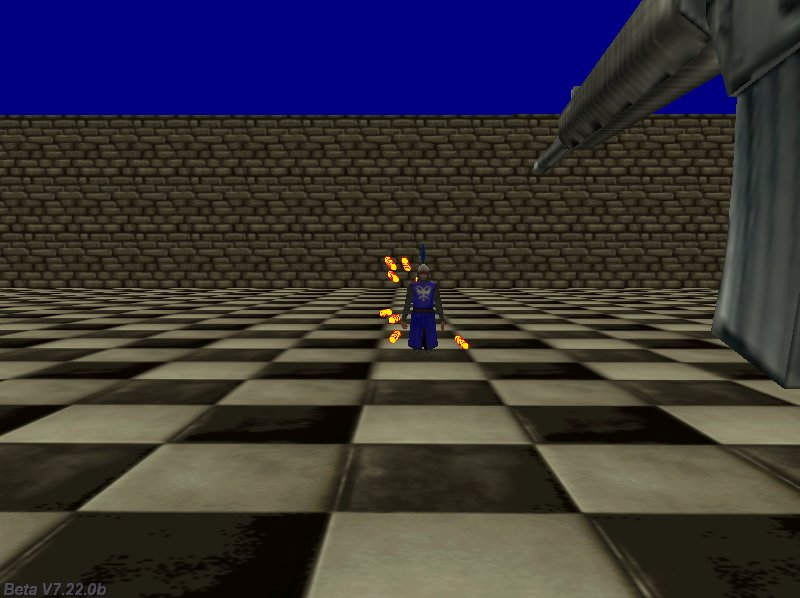Q: I would need the code for a rabbit that can detect obstacles (walls) and can jump over them automatically. A: Here you go.   action wall_jumper() // attach this action to your rabbit model {        VECTOR wall_ahead;        VECTOR temp;        var jumper_height = 0;        var dist_to_ground = 0;        var anim_percentage = 0;        while (my.x < 1200) // jump all the walls until my.x reaches 1200 (play with 1200)        {                // create a vector that is placed 100 quants in front of the rabbit and close to its feet                vec_set (wall_ahead.x, vector (70, 0, -20)); // play with 100 and -20                vec_rotate (wall_ahead.x, my.pan);                vec_add (wall_ahead.x, my.x);                c_move (my, vector(10 * time_step, 0, jumper_height * time_step), nullvector, GLIDE); // 10 = movement speed                anim_percentage += 10 * time_step;                ent_animate(my, "rockrun", anim_percentage, ANM_CYCLE);                if (c_content(wall_ahead.x, 0) == 3) // detected a wall in front of the rabbit?                {                        // then move upwards for as long as there's a wall in front of the rabbit                        jumper_height = 90; // 90 gives the height of the jump, play with it                }                else // no wall is detected? The let's descend (or continue to move as before)                {                        vec_set(temp.x, my.x);                        temp.z -= 10000;                        dist_to_ground = c_trace(my.x, temp.x, IGNORE_PASSABLE);                        // keep the jumper with its feet above the ground and allow a bit of jumping on regular surfaces as well                        if (dist_to_ground > 70) // play with 70                        {                                jumper_height = -dist_to_ground * 0.15 * time_step; // play with 0.15 (sets the descending speed)                        }                }                wait (1);        } }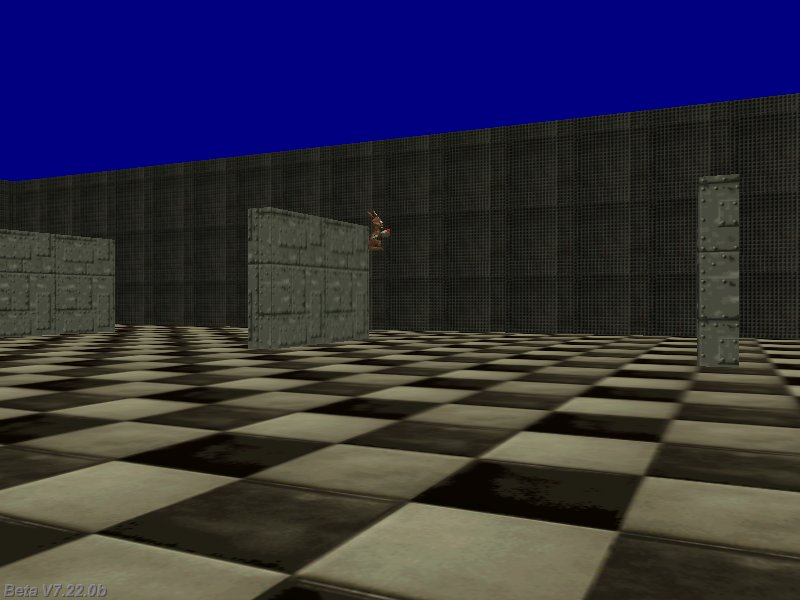Q: How can I detect (inside the enemy code) if the enemy is hit by player's bullets and not by the other enemies' bullets? I need this for my shooter game. A: Set a certain skill for player's bullet to a certain value, and then check for that value inside enemy's event code. Here's an example.   function remove_bullets() {        wait (1);        ent_remove (my); }   function move_bullets() {        VECTOR bullet_speed;        my.emask = ENABLE_IMPACT | ENABLE_ENTITY | ENABLE_BLOCK;        my.event = remove_bullets;        my.pan = you.pan;        my.tilt = you.tilt;        my.skill100 = 999999; // player's bullets will have their skill100 set to 999,999        bullet_speed.x = 35 * time_step;        bullet_speed.y = 0;        bullet_speed.z = 0;        while (my)        {                c_move (my, bullet_speed, nullvector, IGNORE_PASSABLE | IGNORE_YOU);                wait (1);        } }   function got_shot() {        // the event wasn't triggered by player's bullet" (skill100 isn't set to 999,999)? Then nothing should happen!        if (you.skill100 != 999999) {return;}        var anim_percentage = 0;        my.event = NULL;        while (anim_percentage < 100)        {          ent_animate(my, "death", anim_percentage, NULL);      anim_percentage += 3 * time_step;                wait (1);        }        set (my, PASSABLE); }   action my_enemy() {        my.emask = ENABLE_IMPACT | ENABLE_ENTITY;        my.event = got_shot; }   function attach_weapon() {        VECTOR bullet_pos;        set(my, PASSABLE);        while (1)        {                vec_set (my.x, vector (20, -10, 15));                vec_rotate (my.x, you.pan);                vec_add (my.x, you.x);                my.pan = you.pan;                my.tilt = camera.tilt;                if (mouse_left && (total_frames % 10 == 1)) // fire 6 bullets per second at a frame rate of 60                {                        vec_for_vertex(bullet_pos, my, 6);                        ent_create("bullet.mdl", bullet_pos, move_bullets);                }                wait (1);        } }   action players_code() {        fps_max = 60; // limit the frame rate to 60 fps        player = my;        ent_create ("weapon1.mdl", nullvector, attach_weapon);        while (1)        {                c_move (my, vector(10 * (key_w - key_s) * time_step, 6 * (key_a - key_d) * time_step, 0), nullvector, IGNORE_PASSABLE | GLIDE);                vec_set (camera.x, player.x);                camera.z += 30;                camera.pan -= 5 * mouse_force.x * time_step;                camera.tilt += 3 * mouse_force.y * time_step;                player.pan = camera.pan;                wait (1);        } }